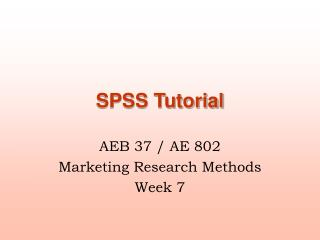Download PresentationSPSS Tutorial

# SPSS Tutorial

Download Presentation## SPSS Tutorial

- - - - - - - - - - - - - - - - - - - - - - - - - - - E N D - - - - - - - - - - - - - - - - - - - - - - - - - - -
##### Presentation Transcript

1. SPSS Tutorial AEB 37 / AE 802 Marketing Research Methods Week 7

2. Cluster analysis Lecture / Tutorial outline • Cluster analysis • Example of cluster analysis • Work on the assignment

3. Cluster Analysis • It is a class of techniques used to classify cases into groups that are relatively homogeneous within themselves and heterogeneous between each other, on the basis of a defined set of variables. These groups are called clusters.

4. Cluster Analysis and marketing research • Market segmentation. E.g. clustering of consumers according to their attribute preferences • Understanding buyers behaviours. Consumers with similar behaviours/characteristics are clustered • Identifying new product opportunities. Clusters of similar brands/products can help identifying competitors / market opportunities • Reducing data. E.g. in preference mapping

5. Steps to conduct a Cluster Analysis • Select a distance measure • Select a clustering algorithm • Determine the number of clusters • Validate the analysis

6. Defining distance: the Euclidean distance Dij distance between cases i and j xkivalue of variable Xkfor case j Problems: • Different measures = different weights • Correlation between variables (double counting) Solution: Principal component analysis

7. Clustering procedures • Hierarchical procedures • Agglomerative (start from n clusters, to get to 1 cluster) • Divisive (start from 1 cluster, to get to n cluster) • Non hierarchical procedures • K-means clustering

8. Agglomerative clustering

9. Agglomerative clustering • Linkage methods • Single linkage (minimum distance) • Complete linkage (maximum distance) • Average linkage • Ward’s method • Compute sum of squared distances within clusters • Aggregate clusters with the minimum increase in the overall sum of squares • Centroid method • The distance between two clusters is defined as the difference between the centroids (cluster averages)

10. K-means clustering • The number k of cluster is fixed • An initial set of k “seeds” (aggregation centres) is provided • First k elements • Other seeds • Given a certain treshold, all units are assigned to the nearest cluster seed • New seeds are computed • Go back to step 3 until no reclassification is necessary Units can be reassigned in successive steps (optimising partioning)

11. Hierarchical vs Non hierarchical methods Hierarchical clustering • No decision about the number of clusters • Problems when data contain a high level of error • Can be very slow • Initial decision are more influential (one-step only) Non hierarchical clustering • Faster, more reliable • Need to specify the number of clusters (arbitrary) • Need to set the initial seeds (arbitrary)

12. Suggested approach • First perform a hierarchical method to define the number of clusters • Then use the k-means procedure to actually form the clusters

13. Elbow rule (2): the scree diagram

14. Validating the analysis • Impact of initial seeds / order of cases • Impact of the selected method • Consider the relevance of the chosen set of variables

15. SPSS Example

16. Number of clusters: 10 – 6 = 4

17. Open the dataset supermarkets.sav From your N: directory (if you saved it there last time Or download it from: http://www.rdg.ac.uk/~aes02mm/supermarket.sav • Open it in SPSS

18. The supermarkets.sav dataset

19. Run Principal Components Analysis and save scores • Select the variables to perform the analysis • Set the rule to extract principal components • Give instruction to save the principal components as new variables

20. Cluster analysis: basic steps • Apply Ward’s methods on the principal components score • Check the agglomeration schedule • Decide the number of clusters • Apply the k-means method

21. Analyse / Classify

22. Select the component scores Untick this Select from here

24. Output: Agglomeration schedule

25. Number of clusters Identify the step where the “distance coefficients” makes a bigger jump

26. The scree diagram (Excel needed)

27. Number of clusters Number of cases 150 Step of ‘elbow’ 144 __________________________________ Number of clusters 6

28. Now repeat the analysis • Choose the k-means technique • Set 6 as the number of clusters • Save cluster number for each case • Run the analysis

29. K-means

30. K-means dialog box Specify number of clusters